Latest Teaching jobs   »   Time And Work- Mathematics Notes For...

# Time And Work- Mathematics Notes For CTET Exam: Free PDFMathematics is an equally important section for CTET, MPTET, KVS & DSSSB Exams and has even more abundant importance in some other exams conducted by central or state govt. Generally, there are questions asked related to basic concepts and properties of the Time And Work.

To let you make the most of Mathematics section, we are providing important facts related to the Time And Work. At least 1-2 questions are asked from Time And Work topic in most of the teaching exams. We wish you all the best of luck to come over the fear of the Mathematics section.

How to Overcome Exam Fever, Especially When You Fear Maths

## TIME AND WORK

Work to be done is usually considered as one unit. It may be constructing a Tower or a School, filling up or emptying a tank or cistern or eating certain amount of food.

There are some basic assumptions that are made in the problems on Time and Work. These are taken for granted and are not specified in every problem.

• If a person does some work in a certain number of days, then we assume that he does the work uniformly, i.e., he does the SAME amount of work every day.

For example, if a person can do some work in 10 days, he does 1/10th of the work in one day.

If a person completes the work in 8 days, he does 1/8th of the work on each day and conversely, if a person can complete 1/8th of the work in one day, he can complete the work in 8 days.

Example: A, B and C contract a work for Rs. 840. Together A and B do  of the work. The share of C should be: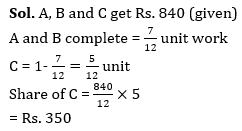If a tap can fill a tank in 40 minutes, then in one minute: It can fill 1/40th part of the tank.

• If there is more than one person carrying out the work, it is assumed that each person unless otherwise specified does the same amount of work each day. This means they share the work equally.

If two people together can do the work in 9 days it means that one man can do it in 18 days. This, in turn means, each person can do 1/18th of the work per day.

If a man works three times as fast as a boy does, the man takes one-third of the time the boy takes to complete the work. If the boy takes 15 days to complete the work, then the man takes 5 days to complete the work.

This method is known as “UNITARY METHOD” i.e. the time taken per “Unit Work” or number of persons required to complete “Unit Work” or work completed by “Unit Person” in “Unit Time“. etc., is what is first calculated.

Example: A and B can do a piece of work in 96 days B and C in 120 days and C and A in 72 days. In how many days all three together can do the work?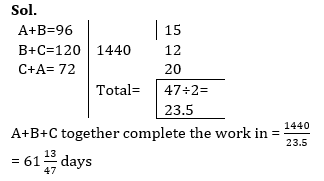Recollect the fundamentals on variation:

• Time remaining constant, Work and Men are directly proportional to each other, i.e., if the
• work increases the number of men required to complete the work in the same number of days increases proportionately and vice-versa.
• Work remaining constant, Men and Days, are inversely proportional, i.e., if the number of men increases, the number of days required to complete the same work decreases in inverse proportion and vice-versa.
• The number of workingmen remaining constant, Work and Days are directly proportional i.e., if the work increases, the number of days required to complete the work with the same number of working men also proportionately increases and vice-versa.

In the concept of MANDAYS, the number of men multiplied by the number of days that they take to complete the work will give the number of mandays required to do the work. The total number of mandays required to complete a specific task will remain a constant. So, if we change one of the variables – men or days – the other will change accordingly so that their product will remain constant. The two variables – men and days – are inversely proportional to each other, when work is constant.

Example: If 15 men take 60 days to complete a job, in how many days can 10 men complete the same job?

Sol: If 15 men can complete the job in 60 days, then the number of mandays required for the work is 15 × 60 = 900 mandays.

If this work is to be done by 10 men, since the number of mandays will be same for same amount of work, the number of days they will take is 900/10 = 90 days.

 If M₁ men can do W₁ work in D₁ days working H₁ hours per day and M₂ men can do W₂ work in D₂ days working H₂ hours per day, then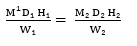Example: A piece of work can be done by 24 men in 4 days working 10 hours a day. The numbers of men are needed to complete another work which is twice as large as the first one in 48 days working 8 hours a day are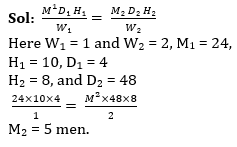PIPES AND CISTERNS

There can be pipes or taps filling or emptying tanks with water. The time taken by different taps to fill or empty the tank may be different. Problems related to these can also be dealt with in the same manner as the foregoing problems on Work have been dealt with.

There is only one difference between the problems on regular Work and those in Pipes and Cisterns. In Pipes and Cisterns, a filling pipe or tap does positive work and an emptying pipe or a leak does negative work.

Example: Two pipes A and B separately can fill an empty tank in 30 minutes and 45 minutes respectively. If both the pipes are opened simultaneously, how long will it take to fill the empty tank?

Sol:

Part of the tank filled by A in 1 minute = 1/30th . Part of the tank filled by B in 1 minute = 1/45th . Part of the tank filled by both A and B in one minute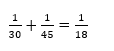The tank can be filled in 18 minutes

Example: A pump can fill a tank in 8 hours. Because of leak in the tank it took 10 hours to fill the tank. If the tank is full, how much time will the leak take to empty it?

Sol.

 A= 8 5 A+B=10 40 4 1

B or empty pipe = 1 unit

Leak pipe take = 40 hours to empty the tank

Example: A pipe can fill a tank in 6 hours. After half the tank is filled, 1 more similar tap is opened. What is the total time taken to fill the tank completely?

Sol. A pipe can fill a tank in= 6 hours

Half tank fill by it= 3 hours

Half tank fill by 2 similar pipes = 1.5 hours

Total time = 3+1.5 = 4.5 hours

•विशेषण- परिभाष�...
•Phases of Teaching - Stages of Teaching ...
•Operant and Classical Conditioning - Dif...
•संज्ञा: परिभाष�...
•अलंकार - परिभाष�...
•Stages of Development- Child Growth and ...